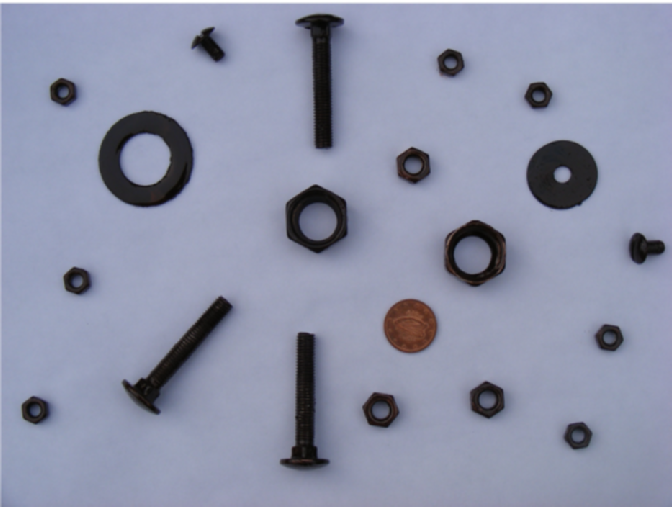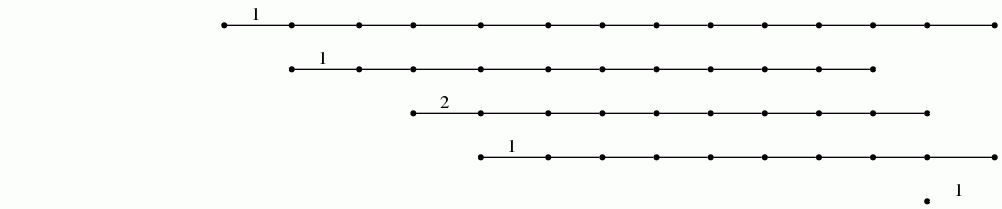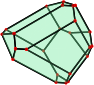Module Content

Data analysis is a process of inspecting, cleansing, transforming, and modeling data with the goal of discovering and communicating useful information, informing conclusions, and supporting decision-making. Data analysis involves many overlapping areas of study, such as descriptive statistics (concerned with summarizing information in a sample), statistical inference (concerned with inferences about a population based on properties of a sample), machine learning (concerned with performing tasks using algorithms that rely on patterns and inference), data mining (sometimes considered a subfield of machine learning and concerned with discovering patterns in large data sets), information visualization (concerned with the visual representations of abstract data to reinforce human cognition), and several other areas. See this interesting review for comments about the related term data science.

Geometry is concerned with questions of shape, size, relative position of figures, isometry, and the properties of space. It underlies much of data analysis, as can be seen from textbooks such as those by [Kendall], [Le Roux], [Kirby], [Tossdorff], [Hartmann], [Outot], [Tierny], [Edelsbrunner], [Patrangenaru], [Biau], [Wichura], [Dryden]. The recent online textbook Mathematical Foundations for Data Analysis by Jeff M. Phillips again emphasizes the importance of a geometric understanding of techniques when applying them to data analysis.

This module focuses on some geometric methods used in data analysis. It covers the geometric and algorithmic aspects of these methods, as well as their implementation as Python code on Linux computers, and their application to a range of different types of data. The first half of the course emphasizes geometric aspects of classical techniques such as least squares fitting, principal component analysis, hierarchical clustering, nearest neighbour searching, and the Johnson-Lindenstrauss Theorem for dimensionality reduction. The second half of the course covers more recent techniques that have been developed over the last two or three decades, and emphasizes topological aspects as well as geometric aspects. The second half of the course makes use of R interfaces to Python Mapper and to efficient C++ routines for persistent homology.

Part I: Classical Techniques (5 ECTS)
• Least Squares Fitting
• Principal Component Analysis
• Hierarchical Clustering and Persistence
• Nearest Neighbours and the Johnson–Lindenstrauss Theorem
Part II: Topological Data Analysis (5 ECTS)
• Topological Preliminaries
• Mapper Clustering
• Persistent Homology
• Fundamental Group

Module Coordinates

• Lecturer: Graham Ellis & Emil Sköldberg
• Lectures:
Fri 14.00pm, ES, ADB1019 (or IT206)
• Tutorials: Wednesday and Friday lectures will often take the format of a tutorial and so no formal tutorials are scheduled.
• Recomended text: Part I is based on chapters from the textbook Multivariate Analysis by Sir Maurice Kendall and chapters from the online textbook Mathematical Foundations for Data Analysis by Jeff M. Phillips . Part II is based on the survey An introduction to Topological Data Analysis: fundamental and practical aspects for data scientists by Frédéric Chazal and Bertrand Michel .
• Problem sheet: available here. (A list of exam-type problems for self-study is available here.)
• Module Website: Information and module documents will be posted to this site, which is linked from the Blackboard MA500 Geometric Foundations of Data Analysis pages. Blackboard will also be used for announcements and for posting grades.

Module Assessment

Part I will be assessed by a 2-hour written exam (52%) and three continuous assessment assignments (16% each).

Part II will be assessed by a 2-hour written exam (50%) and two continuous assessment assignments (25% each).

Each exam will consist of four questions, with full marks for four correct answers.

Each assignment will consist of a data analysis problem that needs to be tackled using the Python programming language, and submitted (by email to both lecturers) as a PDF document.

None so far.

Emil's Lecture Notes

These will be posted here.

Exam Details

A guide to what to expect on the MA500 exams can be found here.

Lecture Notes

 Lecture Notes (Click number to download notes for the lecture.) Lecture Summaries 1 Began by explaining the terms "geometry", "data analysis", "statistics", "probability". Then explained how to find the line y=b0 + b1 x that "best fits" a collection of data points (xi,yi) for i=1,2,...,n. We took "best fit" to mean the line that minimizes the sum of the squares of the residuals ei = yi - b0 - b1xi . 2 Explained how to determine the best (in the least squares sense) plane that fits data points (yi,xi,1 + ... + xi,p-1) in Rp for i=1,2, ..., n. Also explained how to determine the best (in the least squares sense) polynomial of degree at most d that fits data points in R2. Introduced the coefficient of determination R2 = SSR/SSTO . Typically R2 is close to 1 when the fit is good (i.e. when the fit "explains" a lot of the variation in the yi). Next lecture we'll show that 0<= R2 <= 1. We'll also give the formula for R2 in matrix notation. 3 Proved that the coefficient of determination R2 satisfies 0<= R2 <= 1 for p=2. Then gave the matrix notation for computing R2 for p>=2 and stated, without proof, that for p>=2 again 0<= R2 <=1. One often says that "R2 percent of the variation is explained by considering the p-1 independent variables". Gave a method, involving the F-distribution, for choosing between the two hypotheses C1: βi=0 for all i=1,...,p-1. C2: βi is non-zero for at least one i. OOPS!!!! In Lecture 3: on page 4 I give the correct formula SSR=B^rX^tY - n ybar^2 . on page 5 I give the correct formula MSR=SSR/(p-1) But on page 6 I make a silly slip and write MSR=(YtY - BtXtY)/(p-1) instead of MSR=(BtXtY - n ybar2)/(p-1). However, on page 6 the value MSR=26922 is cogged from the book and so should be correct. 4 Explained how to obtain Bonferroni simultaneous confidence intervals for q of the coefficients βk in the regression model. Then started to talk about Principal Component Analysis. (The second homework will use this technique to analyse vectors v1, ..., vn representing n digital images of faces). 5 Explained that the aim of Principal Component Analysis is to find an orthogonal matrix A for which ACAt is diagonal, where C is the covariance matrix of your data points v1, ..., vn in Rp. 6 Gave an example of PCA in gait analysis. Then started towards a proof of the Spectral Theorem. 7 Proved the spectral theorem: any real symmetric nxn matrix has n linearly independent eigenvectors. This is the basis of Principal Component Analysis 8 Gave an introduction to hierarchical cluster analysis, dendrograms, and barcodes. Illustrated these notions through two examples: 1) clustered five objects for which pairwise distances were given; 2) counted the number of objects in the following digital photo, and counted the number of these objects that have holes in them.During the lecture I illustrated dendrroagrams and barcodes using the GAP software system for computational algebra. The code for reproducing the examples is here. 9 Described the Smith-Waterman algorithm for measuring the similarity s(V,W) between two sequences V, W of letters. It finds a maximal scoring local alignment and returns the score. By scaling, we can assume that 0<= s(V,W) <= 1 on any finite set of data. We can then define the dissimilarity measure d(V,W) = 1-s(V,W). In general we do not suppose that d(V,W) satisfies all three axioms of a metric. But when it does we should expect it to be more easily interpreted. Showed the Clustal Omega online resource for determining the (dis)similarity of genetic sequences, and for returning the output in the form of a dendrogram (or phylogenetic tree). 10 Began by explaining how cluster analysis and barcodes can be used to investigate the geometric shape of a data set S in Rn. A computer demonstration was given for a subset of points S in R2 corresponding to the following digital image of a starfish.The corresponding barcode isThen described a single-likage hierarchical clustering algoithm for producing a barcode from the matrix of distances/dissimilarities between n objects. 11 Introduced the nearest neighbour problem through two examples. Then considered a two-step strategy for an efficient solution. Part of this strategy involves Voronoi tessellations of Euclidean space and so ended up talking about Voronoi tessellations and Voronoi regions (with 2-dimensional illustrations). 12 Began with a review of how to construct Voronoi cells as intersetions of half-spaces. Showed the following 3-dimensional Voronoi cell.Described an algorithm, involving Voronoi cells, for finding the closest point in a finite set S to a vector v in Rn, the finite set S being a collection (or database) of points in Rn. Stated the Johnson-Lindenstrauss Theorem and the Norm Preservation Proposition. Next lecture I'll explain how the latter implies the former, and that will complete Part I of the module. 13 Talked more about the Johnson-Lindenstrauss Theorem and the Norm Preservation Proposition. See these notes for more information. END OF PART I OF THE MODULE Started to talk about the paper "Topology based data analysis identifies a subgroup of breast cancers with a unique mutational profile and excellent survival" by Nicolau, Levine and Carlsson. 14 Introduced the notion of a simplicial complex K. Introduced convex hulls in order to explain what is meant by the geometric realisation |K| of a simplicial complex K. Ended up explaining how, from an nxn distance matrix, we can construct a simplicial complex Kε for any choice of threshold ε>0. In this way we can associate a topological space |Kε| to any distance matrix. 15 We considered a sample S of 250 readings x_i=(hi,0, hi,2, hi,4) of water levels at Galway harbour, where hi,0 is the initial level when person i reaches the harbour, hi,2 is the level 2 hours later, and hi,4 is the level 4 hours after the initial reading. We constructed a 250x250 matrix of distances di,j=||xi-xj|| and used this to construct a simplicial complex Kε for various thresholds ε>0. The graph of one of these simplicial complexes was as follows.This graph seems to capture the "cyclic nature" of tides! Repeated this process for a sample of 750 points sampled from two non-intersecting sectors of a torus. In this second example the graph had two connected components, each component having a "circular shape". 16 Stated Leray's nerve theorem: Given an open cover U of a compact space X for which each non-empty intersection of finitely many sets in U is contractible, then the geometric realization |NU| of the nerve of U is homotopy equivalent to the space X. (I forgot to write "compact" in the lecture.) Next lecture we'll use this result to motivate the use of the simplicial complex Kε in data analysis. We'll call Kε the clique complex. 17 Explained how Leray's nerve theorem motivates the use of the clique complex Kε in data analysis. Briefly explained the content of this paper which uses the clique complex Kε to analyse a data base of 8 million 3x3 textons taken from just over 4000 digital photographs of the netherlands. 18 Described the Mapper clustering procedure. Next lecture we'll see some computer examples of this. 19 St Patrick's Day 20 Gave two examples of the Mapper clustering procedure. Then defined the Betti numbers of a simplicial complex \$K\$. Gave an example in which the Betti numbers were calculated for a particular simplicial complex K. 21 Defined the homology Hn(K) of a simplicial complex K (over a field). This is a vector space whose dimension equals the degree n Betti number: βn = dim (Hn(K)) . Explained that an inclusion of simplicial complexes L < K induces a linear homomorphism of vector spaces Hn(L) ---> Hn(K) . Given a matrix of distances between n objects in a dataset, and given an increasing sequence of thresholds, one can construct a sequence of inclusions of clique complexes. For each n this sequence yields a sequence of linear homomorphisms of degree n homology vector spaces. It was explained how the sequence can be represented using barcodes and then used to extract meaningful geometric information about the dataset. The following example from the R-TDA package was explained. library(TDA) Circle1 <- circleUnif(25) Circle2 <- circleUnif(25, r=1.2) Circle3 <- circleUnif(25, r=1.4) Circle4 <- circleUnif(25, r = 2) + 4.5 Circle5 <- circleUnif(25, r = 2.2) + 4.5 Circle6 <- circleUnif(25, r = 2.4) + 4.5 Circles <- rbind(Circle1, Circle2, Circle3, Circle4, Circle5, Circle6) plot(Circles, pch = 16, xlab = "",ylab = "") DiagLim <- 4 maxdimension <- 1 Diag <- ripsDiag(Circles, maxdimension, DiagLim, printProgress = TRUE) par(mfrow = c(1, 1)) plot(Diag[["diagram"]], barcode = TRUE) 22 Defined the persistent Betti numbers of a filtered simplicial complex, and explained how to represent them as barcodes. 23 Tutorial 24 Tutorial